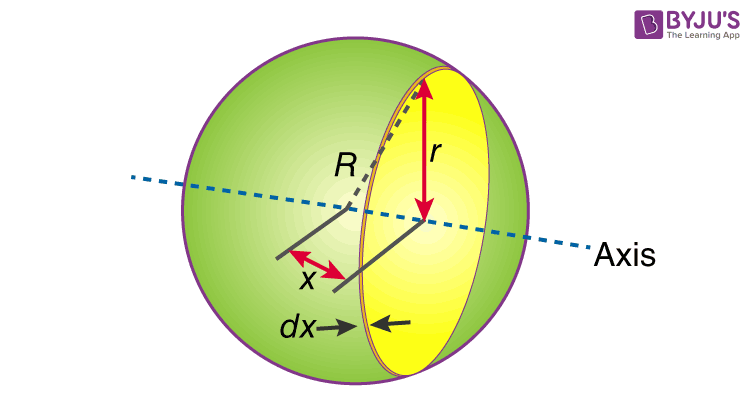Checkout JEE MAINS 2022 Question Paper Analysis : Checkout JEE MAINS 2022 Question Paper Analysis :

# Moment Of Inertia Of Sphere

Moment of inertia of sphere is normally expressed as;

 I = (⅖ )MR2

Here, R and M are the radius and mass of the sphere respectively. Students have to keep in mind that we are talking about the moment of inertia of a solid sphere about its central axis above. Additionally, if we talk about the moment of inertia of the sphere about its axis on the surface it is expressed as;

 I = (7/5) MR2

## Moment Of Inertia Of Sphere DerivationThe moment of inertia of a sphere expression is obtained in two ways.

• First, we take the solid sphere and slice it up into infinitesimally thin solid cylinders.
• Then we have to sum the moments of exceedingly small thin disks in a given axis from left to right.

We will look and understand the derivation below.

First, we take the moment of inertia of a disc that is thin. It is given as;

I = (½ )MR2

In this case, we write it as;

dI (infinitesimally moment of inertia element) = ½ r2dm

Find the dm and dv using;

dm = ρ dv

ρ = density of a thin disk of mass dm

dv = volume of the thin disk

dv = π r2 dx

Now we replace dV into dm. We get;

dm = ρ π r2 dx

And finally, we replace dm with dI.

dI = (½) ρ π r4 dx

The next step involves adding x to the equation. If we look at the diagram we see that r, R and x forms a triangle. Now we will use the Pythagoras theorem which gives us;

r2 = R2 – x2

Now if we substitute the values we get;

dI = ½ ρπ (R2 – x2)2 dx

I = ½ ρ π -RR (R2 – x2)2 dx

After integration and expanding we get;

I =ρ π (8/15) R5

Additionally, we have to find the density as well. For that we use;

ρ = M / V

ρ  = [M / (4/3) πR3]

If we substitute all the values;

I = 8/15 [M / (4/3) πR3] πR5

I = ⅖ MR2

### Parallel Axis theorem